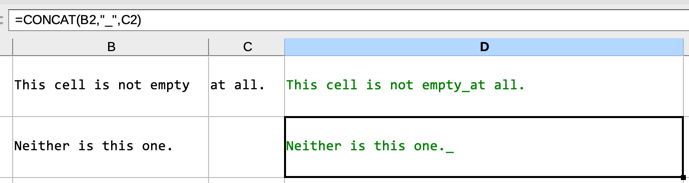# IF function needed (I assume)

In D1, I need to link B1 and C1 with an underscore if C1 is not empty. If C1 is empty I only need B1 in . How can I achieve that?

In the rows of column D, I need to link the cells from column B and those from column C with an underscore. The two cells should only be linked this way if the cell in column C is not empty; if it is empty I need the cell from column B without the one from column C, and without the underscore. How can I achieve this?

The following formula works as expected if the cell in column C has text but not if it has not:

=CONCAT(B1,"_",C1)In this example D1 is perfect but not D2: the content of D2 should be the same as the content of B2 (i.e., without the underscore).

I assume I need an IF function in addition to CONCAT but fail to write it myself to make it work in this context. Any help would be greatly appreciated.

Try

``````=B1 & IF(C1="";;"_" & C1)
``````
1 Like

`=TEXTJOIN("_";1;B1;C1)`.

LibreOffice Help on TEXTJOIN.

Tested on version 7.0.6.2.

Edit: Notice that if B is empty, the formula will show C, not _C.

2 Likes

Something like:
`IF(ISBLANK(C2);C1; C1&"_"&C2)`

1 Like

Thank you! [For the record, I just needed to modify the cell references:]

=IF(ISBLANK(C1),B1, B1&"_"&C1)